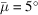International Tables for Crystallography (2006). Vol. C, ch. 2.2, pp. 26-41https://doi.org/10.1107/97809553602060000577

## Contents

• 2.2. Single-crystal X-ray techniques  (pp. 26-41)
• 2.2.1. Laue geometry  (pp. 26-29) | html | pdf |
• 2.2.1.1. General  (pp. 26-27) | html | pdf |
• 2.2.1.2. Crystal setting  (p. 27) | html | pdf |
• 2.2.1.3. Single-order and multiple-order reflections  (pp. 27-29) | html | pdf |
• 2.2.1.4. Angular distribution of reflections in Laue diffraction  (p. 29) | html | pdf |
• 2.2.1.5. Gnomonic and stereographic transformations  (p. 29) | html | pdf |
• 2.2.2. Monochromatic methods  (pp. 29-30) | html | pdf |
• 2.2.2.1. Monochromatic still exposure  (p. 30) | html | pdf |
• 2.2.2.2. Crystal setting  (p. 30) | html | pdf |
• 2.2.3. Rotation/oscillation geometry  (pp. 31-34) | html | pdf |
• 2.2.3.1. General  (p. 31) | html | pdf |
• 2.2.3.2. Diffraction coordinates  (pp. 31-33) | html | pdf |
• 2.2.3.3. Relationship of reciprocal-lattice coordinates to crystal system parameters  (p. 33) | html | pdf |
• 2.2.3.4. Maximum oscillation angle without spot overlap  (pp. 33-34) | html | pdf |
• 2.2.3.5. Blind region  (p. 34) | html | pdf |
• 2.2.4. Weissenberg geometry  (pp. 34-35) | html | pdf |
• 2.2.4.1. General  (p. 34) | html | pdf |
• 2.2.4.2. Recording of zero layer  (p. 34) | html | pdf |
• 2.2.4.3. Recording of upper layers  (pp. 34-35) | html | pdf |
• 2.2.5. Precession geometry  (pp. 35-36) | html | pdf |
• 2.2.5.1. General  (p. 35) | html | pdf |
• 2.2.5.2. Crystal setting  (p. 35) | html | pdf |
• 2.2.5.3. Recording of zero-layer photograph  (p. 35) | html | pdf |
• 2.2.5.4. Recording of upper-layer photographs  (pp. 35-36) | html | pdf |
• 2.2.5.5. Recording of cone-axis photograph  (p. 36) | html | pdf |
• 2.2.6. Diffractometry  (pp. 36-37) | html | pdf |
• 2.2.6.1. General  (p. 36) | html | pdf |
• 2.2.6.2. Normal-beam equatorial geometry  (pp. 36-37) | html | pdf |
• 2.2.6.3. Fixed χ = 45° geometry with area detector  (p. 37) | html | pdf |
• 2.2.7. Practical realization of diffraction geometry: sources, optics, and detectors  (pp. 37-41) | html | pdf |
• 2.2.7.1. General  (p. 37) | html | pdf |
• 2.2.7.2. Conventional X-ray sources: spectral character, crystal rocking curve, and spot size  (pp. 37-38) | html | pdf |
• 2.2.7.3. Synchrotron X-ray sources  (pp. 38-41) | html | pdf |
• 2.2.7.4. Geometric effects and distortions associated with area detectors  (p. 41) | html | pdf |
• References | html | pdf |
• Figures
• Fig. 2.2.1.1. Laue geometry  (p. 27) | html | pdf |
• Fig. 2.2.1.2. A predicted Laue pattern of a protein crystal with a zone axis parallel to the incident, polychromatic X-ray beam  (p. 27) | html | pdf |
• Fig. 2.2.1.3. A multiple component spot in Laue geometry  (p. 28) | html | pdf |
• Fig. 2.2.1.4. The variation with M = λ max min of the proportions of relp's lying on single, double, and triple rays for the case(p. 28) | html | pdf |
• Fig. 2.2.1.5. Geometrical principles of the spherical, stereographic, gnomonic, and Laue projections  (p. 29) | html | pdf |
• Fig. 2.2.3.1. ( a ) Elevation of the sphere of reflection  (p. 30) | html | pdf |
• Fig. 2.2.3.2. Geometrical principles of recording the pattern on ( a ) a plane detector, ( b ) a V -shaped detector, ( c ) a cylindrical detector  (p. 31) | html | pdf |
• Fig. 2.2.3.3. The rotation method. Definition of coordinate systems  (p. 32) | html | pdf |
• Fig. 2.2.3.4. The rotation method. The blind region associated with a single rotation axis  (p. 33) | html | pdf |
• Fig. 2.2.5.1. The screenless precession setting photograph (schematic) and associated mis-setting angles for a typical orientation error when the crystal has been set previously by a monochromatic still or Laue  (p. 35) | html | pdf |
• Fig. 2.2.6.1. Normal-beam equatorial geometry: the angles ω, χ, ϕ, 2θ are drawn in the convention of Hamilton (1974)  (p. 36) | html | pdf |
• Fig. 2.2.6.2. Diffractometry with normal-beam equatorial geometry and angular motions ω, χ and ϕ  (p. 36) | html | pdf |
• Fig. 2.2.7.1. Reflection rocking width for a conventional X-ray source  (p. 38) | html | pdf |
• Fig. 2.2.7.2. Single-crystal monochromator illuminated by synchrotron radiation: ( a ) flat crystal, ( b ) Guinier setting, ( c ) overbent crystal, ( d ) effect of source size (shown at the Guinier setting for clarity)  (p. 39) | html | pdf |
• Fig. 2.2.7.3. Double-crystal monochromator illuminated by synchrotron radiation  (p. 40) | html | pdf |
• Fig. 2.2.7.4. The rocking width of an individual reflection for the case of Fig. 2.2.7.2( c ) and a vertical rotation axis  (p. 40) | html | pdf |
• Tables
• Table 2.2.3.1. Glossary of symbols used to specify quantities on diffraction patterns and in reciprocal space  (p. 32) | html | pdf |
• Table 2.2.5.1. The distance displacement (in mm) measured on the film versus angular setting error of the crystal for a screenless precession () setting photograph  (p. 35) | html | pdf |